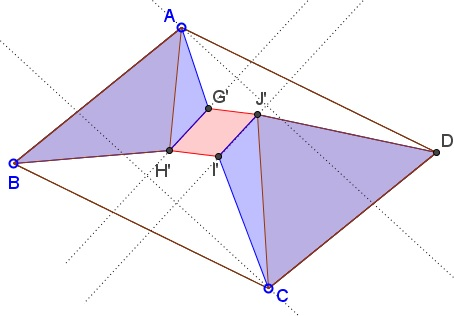# Areas in Two Parallelograms and a Translation

### Problem

$ABCD$ and $GHIJ$ are two parallelograms. $G'H'I'J'$ is a translation of $GHIJ$ and lies entirely within $ABCD.$ Let $[X]$ denote the area of figure $X.$Then $[ABH'G']+[CDJ'I']$ does not depend on the position of $G'H'I'J'$ within $ABCD.$

### Proof

Split the quadrilaterals $ABH'G'$ and $CDJ'I'$ into two triangles as shown.The required result follows from a more basic assertion, namely, that $[\Delta ABH']+[\Delta CDJ']$ does not depend on the position of $G'H'I'J'$ within $ABCD$ and the same holds for $[\Delta AG'H']+[\Delta CI'J'].$ I'll prove the former one first.

Let $h,g$ be the distances from $H'$ to $AB$ and from $J'$ to $CD,$ respectfully. Let $k$ be the distance between two lines (one through $H',$ the other through $J')$ parallel to $AB.$ Then $h+g+k$ equals the distance between $AB$ to $CD.$ And, since $k$ is constant, so is $h+g.$ Now,

$[\Delta ABH']+[\Delta CDJ']=\frac{1}{2}(AB\cdot h+CD\cdot g)=\frac{1}{2}AB(h+g)$

which is constant. Now for the second identity $[\Delta AG'H']+[\Delta CI'J'].$The argument is similar to the foregoing one. Let $r,s,t$ be the lengths of the perpendiculars from $A$ to $G'H',$ from $C$ to $I'J',$ and $t$ the distance between $G'H'$ and $I'J'.$ For fixed directions, $r+s+t$ is constant and so is $r+s.$ On the other hand,

$[\Delta AG'H']+[\Delta CI'J']=\frac{1}{2}(G'H'\cdot r+I'J'\cdot s)=\frac{1}{2}GH(r+s),$

which is constant.

### Remark

When both $ABCD$ and $GHIJ$ are squares the above leads to additional results.

Assume both $ABCD$ and $GHIJ$ are squares:Then:

1. $[\Delta ADG']+[\Delta CBI']=[\Delta ABH']+[\Delta CDJ'],$

2. $[\Delta AG'H']+[\Delta CI'J']=[\Delta BH'I']+[\Delta DG'J'].$

### Extension

The above remains true if the two shapes remain invariant under a rotation by $90^{\circ}.$ For example, Tran Quang Hung has generalized the foregoing claims as illustrated belowandTran Quang Hung has extended the statements to the case of two even sided polygons.

### Acknowledgment

The problem has been posted by Tran Quang Hung at the CutTheKnotMath facebook page.